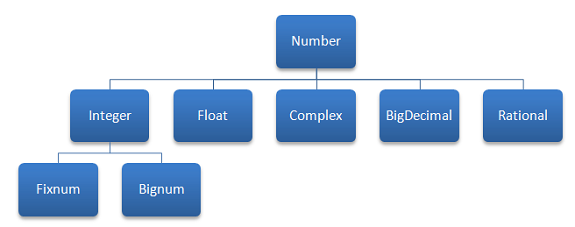# Introduction To Ruby Variables

## Choosing A Name

In Ruby, variables should be written with lower case characters and cannot include spaces or symbols. An underscore can be used to separate words if more than one word is needed to describe what is being stored. The following are reserved words, part of the Ruby language and should not be used as variable names,

 BEGIN END alias and begin break case def defined? do else elsif end ensure false for if module next nil not or redo rescue retry return self super then true undef unless until when while yield

## Data Types

Ruby is a dynamically typed language. That means that the Ruby interpreter works out the data type from the context. You do not need to declare a variable in Ruby or specify its data type as you would in Visual Basic or C#.

## Numbers

The following hierarchy chart shows the different ways that Ruby understands numbers.### Integer

There are two types of integer in Ruby, Fixnum and Bignum. In most cases, Fixnum is used for any whole number (positive or negative) that can be stored in binary with 31 bits. Larger numbers are stored using the Bignum class. Ruby performs this conversion automatically. Operations using Fixnum are substantially quicker than those using Bignum, particularly when the numbers get very large.

The following examples show how you might make a variable store an integer,

```# positive integer age = 42 puts "age" puts age puts age.class puts # negative integer temperature = -15 puts "temperature" puts temperature puts temperature.class puts # integer using separators pocket_cube_positions = 3_674_160 puts "pocket_cube_positions" puts pocket_cube_positions puts pocket_cube_positions.class puts # integer in binary binary_num = 0b1111_1111 puts "binary_num" puts binary_num puts binary_num.class puts # integer in hexadecimal hex_num = 0xFF puts "hex_num" puts hex_num puts hex_num.class puts # integer in octal oct_num = 0377 puts "oct_num" puts oct_num puts oct_num.class puts # larger number cube_positions = 43_252_003_274_489_856_000 puts "cube_positions" puts cube_positions puts cube_positions.class puts gets```

Copy this example to a text file and save as a Ruby program. When you run it, you should get the following output,When you put the variable name in quotes, the interpreter assumes that you want to output the word and not the value stored by the variable name. Any underscores are ignored - they are there to make it easier for a programmer to read the source code. Numbers assigned using different number bases are output in denary. The .class method returns the class that the number belongs to. This is a useful way to find out how Ruby is understanding the data type of your variable.

### Float

Float is named after the method that computers use to store decimal numbers, called floating point numbers. To make a float, you need to make sure that there is a decimal point in the value you assign to a variable and that there are digits either side of the decimal point. The following example illustrates this point,

```# positive float age = 42.5 puts "age" puts age puts age.class puts # negative float temperature = -15.3 puts "temperature" puts temperature puts temperature.class puts # also a float x = 0.5 puts "x" puts x puts x.class puts # one more y = 10.0 puts "y" puts y puts y.class puts gets```

### Complex

The Complex class is used to store complex numbers. A complex number comes in the following form,

a + b i

In the above number, a and b are real numbers. The letter i represents what is called the imaginary part of the number. The letter i stands for the square root of -1.

Some examples of complex numbers are,

```3 + 2i 2.4 + 0.5i 0 + 5i```

The following Ruby example uses complex numbers,

```# 1 + 2i x = Complex(1,2) # 2 + i y = Complex(2,1) # add x and y z = x + y puts z puts z.class gets```

### BigDecimal

Floating point numbers are stored in binary. Whereas the place values to the right of the decimal point in denary are powers of 10, in binary they are powers of 2. This leads to rounding errors. For most cases, the float data type offers sufficient precision, there are some scenarios (like financial calculations) where it is better to use the BigDecimal class.

To make a BigDecimal in Ruby, you write,

`x = BigDecimal("0.1")`

### Rational

A rational number is any number that can be expressed as the quotient of two integers. This means that you can write the number as a fraction a/b where b is not zero. You can do this for any integer, but not all decimal numbers. Some numbers, like the square root of 2, are irrational. When the decimal part of the number is written out, it carries on forever without repeating. PI and e are also irrational numbers.

You can make a Rational in Ruby with,

`r = Rational(1,100)`

This would make the rational number 1/100, or 0.01.

## Strings

The string data type is used to store text - character data. The following example demonstrates several ways to assign strings to variables,

```# single quotes t = 'Some text' tt = 'A single quote, \', displayed inside single quotes' ttt = 'Some text written over more than one line.' puts t puts tt puts ttt puts # double quotes d = "Text in double quotes." dd = "It's easier to use the apostrophe inside double quotes." ddd = "Ruby expression in double quotes - #{d}" puts d puts dd puts ddd gets```

## Constants

Constants are not variables. We use constants when we want to be able to refer to a value by name in our program but know that we won't need to change that value. In Ruby,constants need to begin with a capital letter. For example,

`PI = 3.14`